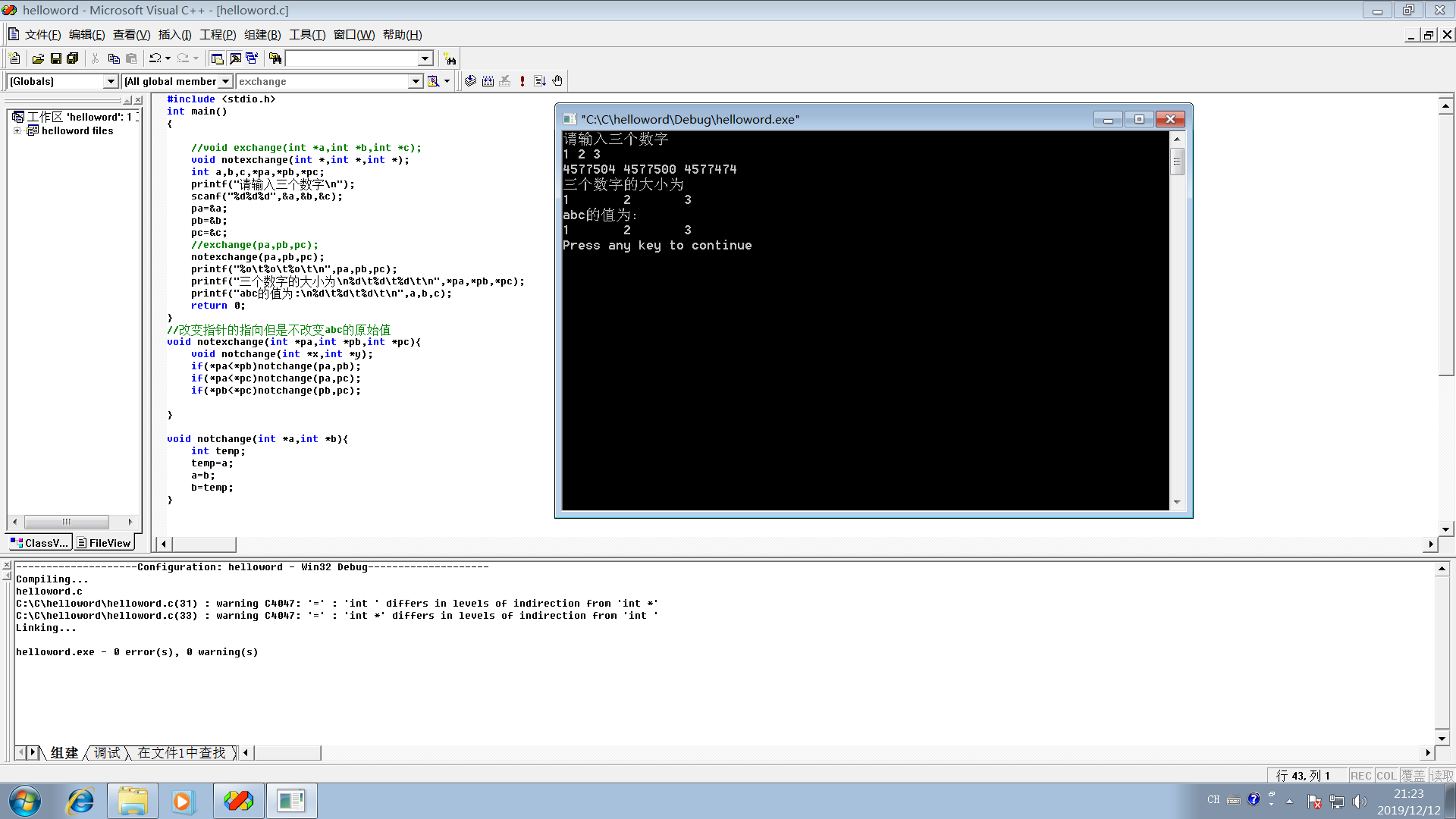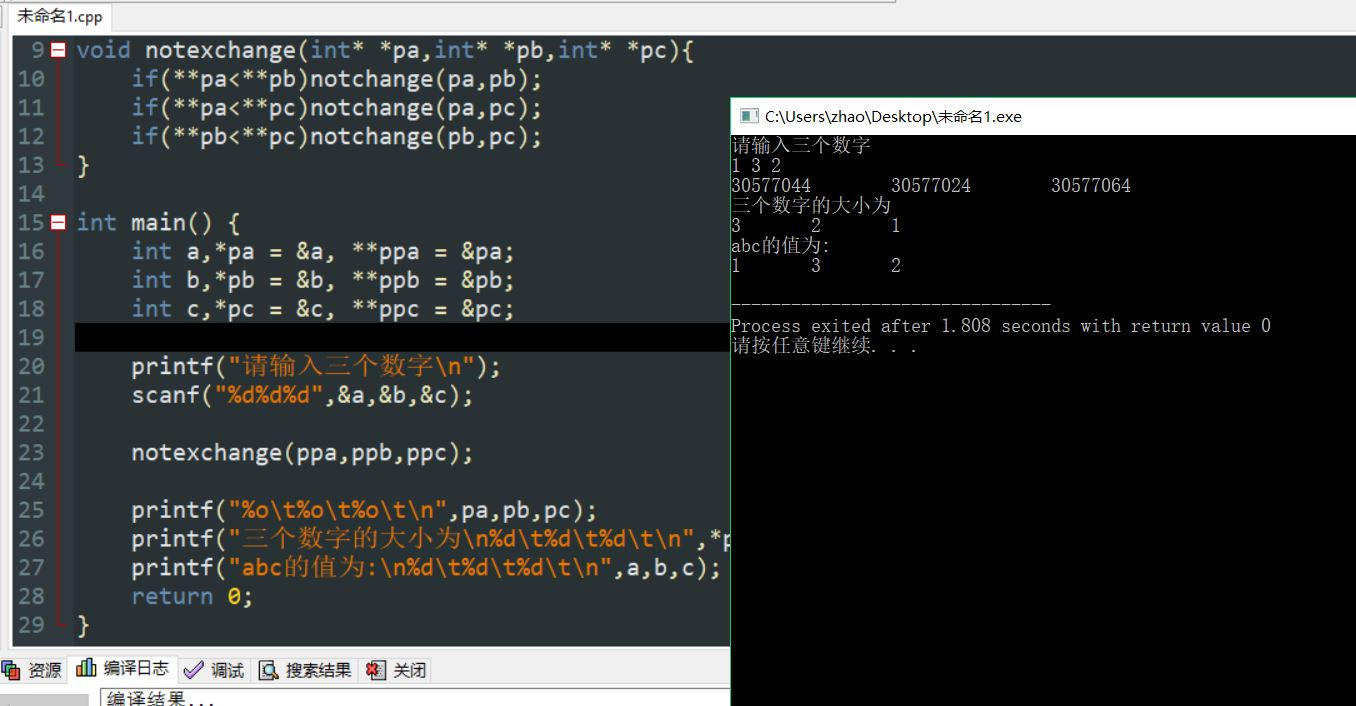2019-12-12 21:42

# c语言怎样更改指针的指向？求大佬解答！

c语言书上的。。。自己没事做，但是- -！脑子有点懵~~

``````#include <stdio.h>
int main()
{
void notexchange(int *,int *,int *);
int a,b,c,*pa,*pb,*pc;
printf("请输入三个数字\n");
scanf("%d%d%d",&a,&b,&c);
pa=&a;
pb=&b;
pc=&c;
notexchange(pa,pb,pc);
printf("%o\t%o\t%o\t\n",pa,pb,pc);
printf("三个数字的大小为\n%d\t%d\t%d\t\n",*pa,*pb,*pc);
printf("abc的值为:\n%d\t%d\t%d\t\n",a,b,c);
return 0;
}
``````

``````void notexchange(int *pa,int *pb,int *pc){
void notchange(int *x,int *y);
if(*pa<*pb)notchange(pa,pb);
if(*pa<*pc)notchange(pa,pc);
if(*pb<*pc)notchange(pb,pc);
}
``````

``````void notchange(int *a,int *b){
int temp;
temp=a;
a=b;
b=temp;
}
``````• 写回答
• 好问题 提建议
• 追加酬金
• 关注问题
• 邀请回答

#### 2条回答默认 最新

•JonathanYTR 2019-12-12 22:18
最佳回答

如果想在函数内修改函数外的值，只能通过传入其指针或引用来修改，你要求的是原值不变指针改变，那传入的就应该是指针的指针。

``````#include <stdio.h>

void notchange(int* *a,int* *b){
int* temp = *a;
*a = *b;
*b = temp;
}

void notexchange(int* *pa,int* *pb,int* *pc){
if(**pa<**pb)notchange(pa,pb);
if(**pa<**pc)notchange(pa,pc);
if(**pb<**pc)notchange(pb,pc);
}

int main() {
int a,*pa = &a, **ppa = &pa;
int b,*pb = &b, **ppb = &pb;
int c,*pc = &c, **ppc = &pc;

printf("请输入三个数字\n");
scanf("%d%d%d",&a,&b,&c);

notexchange(ppa,ppb,ppc);

printf("%o\t%o\t%o\t\n",pa,pb,pc);
printf("三个数字的大小为\n%d\t%d\t%d\t\n",*pa,*pb,*pc);
printf("abc的值为:\n%d\t%d\t%d\t\n",a,b,c);
return 0;
}
``````ide换了吧，用devc++，vs2017都行，vc看着太难受了

评论
解决 无用
打赏 举报